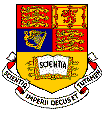Imperial College OF SCIENCE, TECHNOLOGY AND MEDICINE
University of London

Department of Electrical & Electronic Engineering

Electrical & Electronic Eng. (EEE)        BEng, MEng 1st Year
Information Systems Eng. (ISE)
BEng, MEng 1st Year

Digital Electronics 1 (2007-8)

##Objectives

• To impart to you a formalism of logic enabling you to analyse logical processes
• To enable you to implement simple logical operations using combinational logic circuits
• To enable you to understand common forms of number representation in digital electronic circuits and to be able to convert between different representations
• To enable you to understand the logical operation of simple arithmetic and other MSI circuits (Medium Scale Integrated Circuits)
• To impart to you the concepts of sequential circuits enabling you to analyse sequential systems in terms of state machines
• To enable you to implement synchronous state machines using flip-flops

Textbook

Lecture Notes (Notes and problem sheets will be added after each lecture)

Lecture 1  Overview (Problem Sheet 1, Solution)
Lecture 2  Introduction to Data Representation (Problem Sheet 2, Solution)
Lecture 3  Boolean Algebra and Combination Logic 1 (Problem Sheet 3, Solution)
Lecture 4  Boolean Algebra and Combination Logic 2 (Problem Sheet 4, Solution)
Lecture 5  Combinational Logic Gates and Implementation  (Problem Sheet 5, Solution)
Lecture 6  More Gates and Multiplexers (Problem Sheet 6, Solution)
Lecture 7  Signed Numbers & Arithmetic Circuits (Problem Sheet 7, Solution)
Lecture 8  Programmable Logic Devices (Problem Sheet 8, Solution)
Lecture 9
Flip-flops & Sequential Circuits (Problem Sheet 9&10, Solution)
Lecture 10 More flip-flops (No problem sheet, same as Sheet 9)
Lecture 11 Counters (Problem Sheet 11, Solution)
Lecture 12 Finite State Machines (Problem Sheet 12, Solution)
Lecture 13 Application Examples (Problem Sheet 13, Solution)